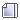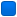###Author Topic: How can I test a numeric string overflow?  (Read 4591 times)

####PaoloC13##### How can I test a numeric string overflow?
« on: May 02, 2020, 01:30:36 am »
Hi to everybody!
I found myself facing a problem: having a string representing a numeric value, how can I define whether or not it oveflows the range of a specific type? (natively, without using any library!)

The answer I gave myself, lies below. In this example I used the type unsigned int. I would ask if anyone had a simpler and more efficient way.

thanks

Code: [Select]
`#include <stdlib.h>#include <stdbool.h>#define _SKIP_LEADING_ZEROES(s) while ( *s == '0' ) s++;#define ISDIGIT(c) ( c > '/' && c < ':' ) // Only numeric chars.#define UINT_MAX_DIGITS 10 // undigned int max. 4294967295 (10 digits).#define ASCII_0 48#define ASCII_1 49#define ASCII_2 50#define ASCII_3 51#define ASCII_4 52#define ASCII_5 53#define ASCII_6 54#define ASCII_7 55#define ASCII_8 56#define ASCII_9 57inline size_t NumLenA(const char *restrict s){ size_t start = (size_t)s; while( ISDIGIT(*s) ) s++; return (size_t)s - start;}bool UIntOverflowA(const char *p){ _SKIP_LEADING_ZEROES(p) // It may be 00000123456789; size_t len = NumLenA(p); if( len > UINT_MAX_DIGITS ) return true; if( len == UINT_MAX_DIGITS ) { if( p > ASCII_4 ) return true; if( p == ASCII_4 ) { if( p > ASCII_2 ) return true; if( p == ASCII_2 ) { //if( p > ASCII_9 ) return true; if( p == ASCII_9 ) { if( p > ASCII_4 ) return true; if( p == ASCII_4 ) { //if( p > ASCII_9 ) return true; if( p == ASCII_9 ) { if( p > ASCII_6 ) return true; if( p == ASCII_6 ) { if( p > ASCII_7 ) return true; if( p == ASCII_7 ) { if( p > ASCII_2 ) return true; if( p == ASCII_2 ) { //if( p > ASCII_9 ) return true; if( p == ASCII_9 ) { if( p > ASCII_5 ) return true; } } } } } } } } } } return false;}`

####frankie

• Global Moderator
• Member
••• Posts: 1941##### Re: How can I test a numeric string overflow?
« Reply #1 on: May 02, 2020, 11:36:46 am »
Try:
Code: [Select]
`bool UIntOverflow(const char *p){ uint32_t v = 0; while(*p && *p>='0' && *p<='9') { uint32_t tmp = v; v = (v*10) + (uint32_t)(*p-'0'); if (tmp > v) return false; //Overflow } return true;}`
« Last Edit: May 02, 2020, 11:46:23 am by frankie »

####PaoloC13##### Re: How can I test a numeric string overflow?
« Reply #2 on: May 02, 2020, 11:26:39 pm »
Hi frankie, thanks for your smart tip. It doesn't seem to work for me, but I tried to fix it:

Code: [Select]
`bool UIntSafeStr(const char *p){ uint32_t v = 0; uint32_t w = 0; while ( *p >= '0' && *p <= '9' ) // (*p != 0) inclusive. { if ( v > 429496729 ) // Test for v *= 10; overflow. return false; v *= 10; w = (unsigned)(*p++ - '0'); if ( UINT_MAX - v < w ) // Test for addition overflow. return false; v += w; } return true;}UIntSafeStr("4294967295"); // Ok!UIntSafeStr("4294967296"); // Overflow!`

« Last Edit: May 02, 2020, 11:31:22 pm by PaoloC13 »

####frankie

• Global Moderator
• Member
••• Posts: 1941##### Re: How can I test a numeric string overflow?
« Reply #3 on: May 03, 2020, 01:01:51 am »
I should have fixed it at last.
Added increment of string pointer and added a check for shifting overflow.
Try it.
Code: [Select]
`#include <stdio.h>#include <stdint.h>#include <stdbool.h>bool UIntOverflow(const char *p){ uint32_t v = 0; while(*p && *p>='0' && *p<='9') { if (v & 0x80000000) //if last bit set return false; //result will overflow uint32_t tmp = v; v = (v * 10) + (uint32_t)(*p++ -'0'); if (tmp > v) return false; //Overflow } return true;}int main(int argc, char *argv[]){ char *str1 = "4294967295"; char *str2 = "4294967296"; printf("%s => %s\n", str1, UIntOverflow(str1) ? "Ok" : "Overflow"); printf("%s => %s\n", str2, UIntOverflow(str2) ? "Ok" : "Overflow"); return 0;}`
« Last Edit: May 04, 2020, 05:12:09 pm by frankie »

####PaoloC13##### Re: How can I test a numeric string overflow?
« Reply #4 on: May 04, 2020, 02:46:02 am »
Great! It works properly.
Honestly, I don't understand the need of the "if ( v & 0x80000000 )" check. I was unable to find an input (to the function) that causes this exit.

At the end, it seems to work well even without it, which is your previous solution.

EDIT:
Quote
4294967295 => Ok
8589934590 => Ok
Press any key to continue...
« Last Edit: May 04, 2020, 03:11:45 am by PaoloC13 »

####frankie

• Global Moderator
• Member
••• Posts: 1941##### Re: How can I test a numeric string overflow?
« Reply #5 on: May 04, 2020, 03:08:57 pm »
Remove the test of last bit set, and try the function for "42949672959"...
Then put back the test before use it in your code!The check prevents a wraparound that could result on an overflowed value still satisfying the check 'tmp > v'.
Checking last bit reports future overflow before multiplication (just doubling it gives an overflow).
« Last Edit: May 04, 2020, 03:28:01 pm by frankie »

####John Z

• Member
•• Posts: 533##### Re: How can I test a numeric string overflow?
« Reply #6 on: May 04, 2020, 03:36:34 pm »
if you are only interested in a limited case of unsigned int 32, then send the incoming string to a Larger storage variable then compare to int32_max.  Check string length less than 11 chars (because if more then alreay too large), then atoll(p) to a long long variable then check if > 4294967295 or int_max.  Can generalize ot other sizes if passing size specifier along with string pointer.
Ends up simply
check length
convert to larger storge
compare to max

####frankie

• Global Moderator
• Member
••• Posts: 1941##### Re: How can I test a numeric string overflow?
« Reply #7 on: May 04, 2020, 04:02:00 pm »
I understood that he don't want use any library function and operate natively.
Maybe also the use of larger storage should be avoided?

####John Z

• Member
•• Posts: 533##### Re: How can I test a numeric string overflow?
« Reply #8 on: May 04, 2020, 04:28:04 pm »
Ah, sorry I see that now.... "(natively, without using any library!)"

####PaoloC13##### Re: How can I test a numeric string overflow?
« Reply #9 on: May 04, 2020, 08:30:44 pm »
Remove the test of last bit set, and try the function for "42949672959"...
Then put back the test before use it in your code!Yes, it was the case I was looking for and that I had missed. Thanks.
But then, I found other cases that provides an exception, for example:

UIntOverflow("8589934590"); // No owerflow!

####PaoloC13##### Re: How can I test a numeric string overflow?
« Reply #10 on: May 04, 2020, 08:44:40 pm »
Maybe also the use of larger storage should be avoided?
I'd say, no, I would just like to practice in speed performances without ready-made things that I can't see how they are coded inside.

####frankie

• Global Moderator
• Member
••• Posts: 1941##### Re: How can I test a numeric string overflow?
« Reply #11 on: May 04, 2020, 09:54:27 pm »
Maybe also the use of larger storage should be avoided?
I'd say, no, I would just like to practice in speed performances without ready-made things that I can't see how they are coded inside.
The problem again is to trace eventual overflow at each stage, the check for the last bit wasn't enough.
Last fix could be:
Code: [Select]
`bool UIntOverflow(const char *p){ uint32_t v = 0; while(*p && (*p>='0' && *p<='9')) { if (v & 0xe0000000) //if last 3 bits are set multiply return false; //by 8 or by 2 will overflow uint32_t tmp = v; v <<= 3; if ((v & 0x80000000) && (tmp & 0xc0000000)) return false; //sum with doubled value will overflow v += tmp << 1; //v*10 done as v*8 + v*2 v += (uint32_t)(*p++ -'0'); //now add next digit if (tmp > v) return false; //Overflow } return true;}`
Anyway if the size of storage isn't relevant a more simple form is the following:
Code: [Select]
`bool UIntOverflow(const char *p){ uint64_t v = 0; while(*p && (*p>='0' && *p<='9')) { v = (v * 10) + (uint64_t)(*p++ -'0'); if (v > 4294967295) return false; //Overflow } return true;}`Last, for our friends who likes more the assembler, this is a very simple routine in assembler having access to the carry bit.
« Last Edit: May 04, 2020, 09:58:24 pm by frankie »

####PaoloC13##### Re: How can I test a numeric string overflow?
« Reply #12 on: May 05, 2020, 02:19:24 pm »
Ok frankie, great work!The last one is the most immediate but also the most performing (if we consider 64 bit processors).

The previous one is a masterpiece of bitwise understanding. I will take the time to go deeper into it. I found it is 1.37 (averagely) slower than the last one.

My fix called UIntSafeStr() I found it is 1.12 (averagely) slower than the last one.

Thanks

####John Z

• Member
•• Posts: 533##### Re: How can I test a numeric string overflow?
« Reply #13 on: May 05, 2020, 09:32:09 pm »
In addition, if you have verified the length of the string before calling this procedure you can speed this up more. There is no need to check the value of v after every digit.  Check it once after all digits have been added.

####frankie

• Global Moderator
• Member
••• Posts: 1941##### Re: How can I test a numeric string overflow?
« Reply #14 on: May 05, 2020, 10:43:58 pm »
Thanks Paolo.
Just for fun I made it in assember 32bits:
Code: [Select]
`bool UIntOverflowAsm(const char *p){ __asm { xor ebx,ebx; mov edx, p;repeat: movsx eax,byte ptr [edx]; cmp eax,0x30; //'0' jb  success; cmp eax,0x39; //'9' ja  success; sub eax,0x30; //*p-'0' shl ebx,1; //ebx = val*2 jc  ovflow; //jump if overflow add eax, ebx; //eax=val*2+actual digit jc  ovflow; //jump if overflow shl ebx,1; //ebx=val*4 jc  ovflow; //jump if overflow shl ebx,1; //ebx=val*8 jc  ovflow; //jump if overflow add ebx,eax; //ebx=val*8+val*2+actual digit=val*10+actual digit jc  ovflow; //jump if overflow inc edx; //p++ jmp repeat; }ovflow: return 0;success: return 1;}`For 64 bits the C short version is already optimized.
As suggested computing the length of the string can shorter the test, but, apart the time to compute length, the string must not include non numeric characters. I.e. testing for "0 foo bar foo" will fail.